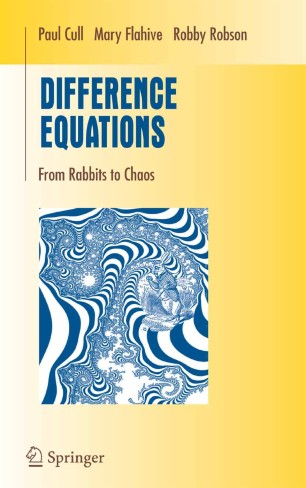# Difference Equations

## From Rabbits to Chaos

• Paul Cull
• Mary Flahive
• Robby RobsonTextbook

Part of the Undergraduate Texts in Mathematics book series (UTM)

1. Front Matter
Pages i-xiii
2. Pages 1-10
3. Pages 11-31
4. Pages 33-65
5. Pages 67-99
6. Pages 101-135
7. Pages 137-178
8. Pages 179-216
9. Pages 217-251
10. Pages 253-295
11. Pages 297-336
12. Back Matter
Pages 337-392

### Introduction

Difference equations are models of the world around us. From clocks to computers to chromosomes, processing discrete objects in discrete steps is a common theme. Difference equations arise naturally from such discrete descriptions and allow us to pose and answer such questions as: How much? How many? How long? Difference equations are a necessary part of the mathematical repertoire of all modern scientists and engineers.

In this new text, designed for sophomores studying mathematics and computer science, the authors cover the basics of difference equations and some of their applications in computing and in population biology. Each chapter leads to techniques that can be applied by hand to small examples or programmed for larger problems. Along the way, the reader will use linear algebra and graph theory, develop formal power series, solve combinatorial problems, visit Perron—Frobenius theory, discuss pseudorandom number generation and integer factorization, and apply the Fast Fourier Transform to multiply polynomials quickly.

The book contains many worked examples and over 250 exercises. While these exercises are accessible to students and have been class-tested, they also suggest further problems and possible research topics.

Paul Cull is a professor of Computer Science at Oregon State University. Mary Flahive is a professor of Mathematics at Oregon State University. Robby Robson is president of Eduworks, an e-learning consulting firm. None has a rabbit.

### Keywords

Finite Matrix algebra difference equation equation function graph theory linear algebra mathematics

#### Authors and affiliations

• Paul Cull
• 1
• Mary Flahive
• 2
• Robby Robson
• 3
1. 1.Dept. Computer Science Dearborn HallOregon State UniversityCorvallisUSA
2. 2.Dept. Mathematics Kidder HallOregon State UniversityCorvallisUSA
3. 3.EduworksCorvallisUSA

### Bibliographic information

• DOI https://doi.org/10.1007/0-387-27645-9